Annulus

Calculate the area of two circles annulus k1 (S, 3 cm) and k2 (S, 5 cm).

Result

S =  50.27 m2

Solution:Leave us a comment of example and its solution (i.e. if it is still somewhat unclear...):Be the first to comment!Next similar examples:

1. Annulus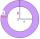Two concentric circles form an annulus of width 10 cm. The radius of the smaller circle is 20 cm. Calculate the content area of annulus.
2. AnnulusThe radius of the larger circle is 8cm, the radius of smaller is 5cm. Calculate the contents of the annulus.
3. Star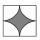Calculate the content of the shaded part of square with side 28 cm.
4. 22/7 circleCalculate approximately area of a circle with radius 20 cm. When calculating π use 22/7.
5. CableCable consists of 8 strands, each strand consists of 12 wires with diameter d = 0.5 mm. Calculate the cross-section of the cable.
6. Circle - simpleCalculate the area of a circle in dm2, if its circumference is 31.4 cm.
7. Pipe cross sectionThe pipe has an outside diameter 1100 mm and the pipe wall is 100 mm thick. Calculate the cross section of this pipe.
8. Circle - easy 2The circle has a radius 6 cm. Calculate:
9. 6-gon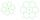Perimeter of regular hexagon is 113. Calculate its circumradius (radius of circumscribed circle).
10. SidewalkThe city park is a circular bed of flowers with a diameter of 8 meters, around it the whole length is 1 meter wide sidewalk . What is the sidewalk area?
11. CD disc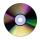A compact disc has a diameter of 11.8 cm. What is the surface area of the disc in square centimeters?
12. Diameter to areaFind the area of a circle whose diameter is 26cm.
13. LawnsBefore a sports hall are two equally large rectangular lawns, each measuring 40 m and 12 m. Maintenance of 10m² lawn cost 45 CZK yearly. On each lawn is circular flowerbed with a diameter 8 meters. How much money is needed each year to take on lawn care?.
14. Two circlesTwo circles with a radius 4 cm and 3 cm have a center distance 0.5cm. How many common points have these circles?
15. WellRope with a bucket is fixed on the shaft with the wheel. The shaft has a diameter 50 cm. How many meters will drop bucket when the wheels turn 15 times?
16. Complementary angles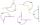Two angles are complementary. If one angle measures 10°, what is the measure of the second angle?
17. The lowestThe lowest temperature on Thursday was 20°C. The lowest temperature on Saturday was 12°C. What was the difference between the lowest temperatures?